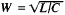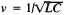# Long Line

(redirected from Long-line)
The following article is from The Great Soviet Encyclopedia (1979). It might be outdated or ideologically biased.

## Long Line

an electrical line formed by two parallel current conductors whose length is greater than the wavelength of the electromagnetic oscillations being transmitted and that are separated by a distance considerably less than the wavelength. A long line is a system with distributed constants (parameters), since every element of its length has at the same time certain values for the inductance L and resistance R of the wires and capacitance C and conductance G between them. The main characteristics of a long line, the characteristic impedance W and the propagation velocity v of electromagnetic waves along it, are governed by these parameters. The instantaneous values for the strength and voltage of an alternating current at any point on a long line are mathematically related by the so-called telegraph equations. A long line is called homogeneous if its parameters are constant over its entire length; if there are no electrical losses in it—that is, if R’ = G = 0 (usually at radio frequencies)—thenand.

In the general case, the input impedance of a long line is complex in nature (it includes resistive and reactive components) and is a function of the length and the type of electrical load at its end (output). The input impedance of an infinitely long line is equal to W. For the maximum transmission of power from a source to the line its input impedance should be resistive and equal to the internal resistance of the source—that is, it must be matched. A long line can operate in three modes: the traveling-wave mode, in which the transmitted power is completely absorbed by the load (the load impedance is resistive and equal to W); the standing-wave mode, in which the transmitted power is completely reflected from the end of the line toward the source (a long line that is short-circuited or open-circuited at the end); and the intermediate mode (the load impedance is complex and is not equal to W).

Long lines are used to transmit information in long-distance telegraph and telephone communications systems, in television, and in radar, as well as to transmit power over wire for great distances.

### REFERENCES

Garnovskii, N. N. Teoreticheskie osnovy elektroprovodnoi sviazi, part 2. Moscow, 1959.
Gonorovskii, I. S. Radiotekhnicheskie tsepi i signaly, part 2. Moscow, 1967.
IU. B. LIUBCHENKO

## Long Line

gear used for catching mainly predatory fish, a type of hooked tackle. A long line consists of a strong rope to which shorter lines with baited hooks are attached.